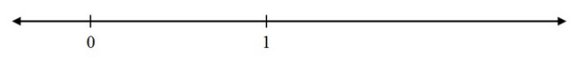• 10/31/13   |   Adjusted: 08/01/18   |   1 file# Find 1/4 Starting from 1, Assessment Variation

• Description
• Files

Mathematically:

• Addresses standards: 3.NF.A.2a3.NF.A.1, and MP.2
• Helps students understand fractions in relation to whole numbers (3.NF.A.2a)
• Requires students to understand that the fraction $\frac{1}{4}$ is formed by partitioning the whole (1) into 4 equal parts (3.NF.A.1)
• Uses a number line to demonstrate students' understanding of fractions as numbers
• Encourages students to think carefully about the placement of the unit fraction $(\frac{1}{4})$ as $0$ and $1$ are arbitrarily placed on the number line

In the classroom:

• Can lead into related discussions such as, "Find $x$ starting from $y$", where $x$ and $y$ are fractions
• Allows students to demonstrate conceptual understanding through a brief conceptual problem with low computational difficulty
• Provides an example of how students might encounter such a task in a computer-based environment

This task was designed to include specific features that support access for all students and align to best practice for English Language Learner (ELL) instruction. Go here to learn more about the research behind these supports. This lesson aligns to ELL best practice in the following ways:

• Provides opportunities for students to practice and refine their use of mathematical language.
• Allows for whole class, small group, and paired discussion for the purpose of practicing with mathematical concepts and language.
• Includes a mathematical routine that reflects best practices to supporting ELLs in accessing mathematical concepts.
• Provides opportunities to support students in connecting mathematical language with mathematical representations.
• Prompts teachers to write essential ideas/concepts/language on the board as a reference for students

• Making the Shifts

How does this task exemplify the instructional Shifts required by CCSSM?

 Focus Belongs to the major work of third grade Coherence Integrates fractions with whole numbers, and lays groundwork for grade 4 fraction expectations; Expands understanding of whole numbers on the number line from previous grades Rigor Conceptual Understanding: primary in this task Procedural Skill and Fluency: not targeted in this task Application: not targeted in this task

The number line below shows two numbers, 0 and 1.Where is $\frac{1}{4}$ on this number line?

• Illustrative Mathematics Commentary and Solution

Commentary:

This task is part of a joint project between Student Achievement Partners and Illustrative Mathematics to develop prototype machine-scorable assessment items that test a range of mathematical knowledge and skills described in the CCSSM and begin to signal the focus and coherence of the standards.

This is the first of three summative assessment tasks for 3.NF.A.2 that progress in difficulty. Each requires that students "understand a fraction as a number on the number line" and "represent fractions on a number line diagram." Part (a) of the standard is about representing unit fractions and part (b) is about representing fractions in terms of unit fractions. The first task involves (a); the second two involve both (a) and (b) at different levels of complexity. The tasks require attention to the whole when thinking about fractions; on a number line, the whole is the interval from 0 to 1.

Mathematical Content:

Standard 3.NF  Find 1/4 starting from 1 asks students to construct a unit fraction on the number line given the unit interval.

Solution:

As part of an assessment, this would be a one-point task; the correct answer is shown in the video.Students will get full credit as long as the point touches the correct tick mark at all.

Mathematical Practices:

Proficiency with the number line is an important component of students’ ability to reason abstractly and quantitatively (MP.2). While the number line is an important tool, these tasks do not ask for strategic use of tools (MP.5) because the number line is provided. An item calling for MP 5 might be one that is easy if the student uses a number line, but difficult if not.